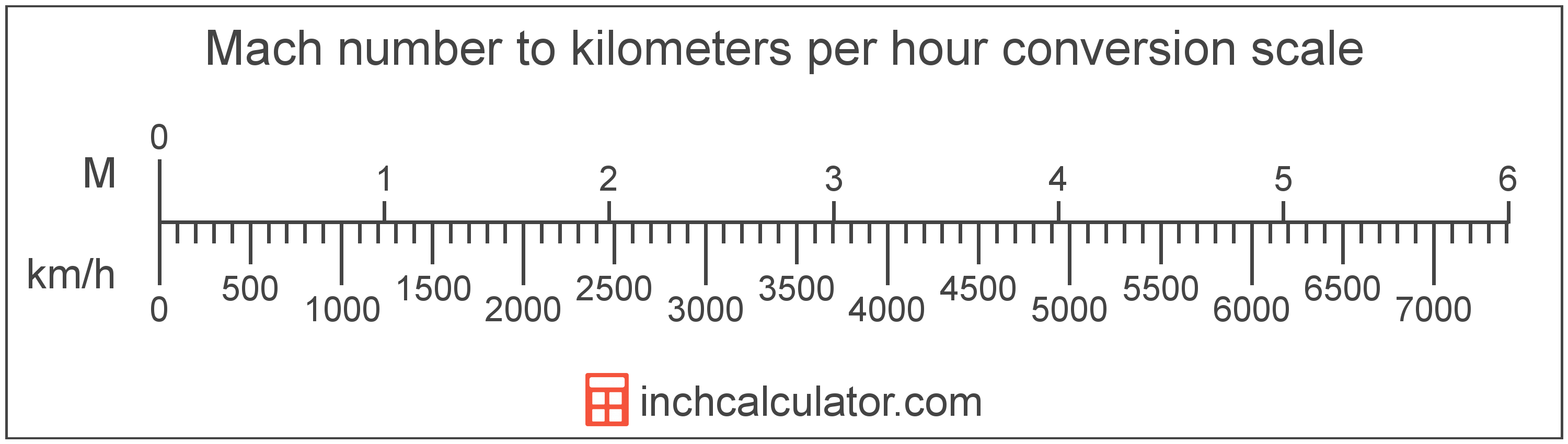# Kilometers Per Hour to Mach Number Conversion

Enter the speed in kilometers per hour below to get the value converted to Mach number.

Results in Mach Number:1 km/h = Mach 0.00081

## How to Convert Kilometers Per Hour to Mach NumberTo convert a kilometer per hour measurement to a Mach measurement, multiply the speed by the conversion ratio. One kilometer per hour is equal to Mach 0.00081, so use this simple formula to convert:

Mach number = kilometers per hour × 0.00081

The Mach number is equal to the speed in kilometers per hour multiplied by 0.00081.

For example, here's how to convert 5 kilometers per hour to Mach number using the formula above.
5 km/h = (5 × 0.00081) = Mach 0.004049

Kilometers per hour and Mach number are both units used to measure speed. Keep reading to learn more about each unit of measure.

## Kilometers Per Hour

Kilometers per hour are a measurement of speed expressing the distance traveled in kilometers in one hour.

The kilometer per hour, or kilometre per hour, is an SI unit of speed in the metric system. Kilometers per hour can be abbreviated as km/h, and are also sometimes abbreviated as kph. For example, 1 kilometer per hour can be written as 1 km/h or 1 kph.

In formal expressions, the slash, or solidus (/), is used to separate units used to indicate division in an expression.

Kilometers per hour can be expressed using the formula:
vkm/h = dkmthr

The velocity in kilometers per hour is equal to the distance in kilometers divided by time in hours.

## Mach Number

Mach, sometimes also referred to as a Mach number, is the ratio of the speed of an object to the speed of sound.

Mach can be abbreviated as M, and is also sometimes abbreviated as Ma. For example, Mach 1 can be written as 1 M or 1 Ma.

Although Mach can be abbreviated M or Ma, the Mach number is typically expressed with the number after the unit name. For example, the expression Mach 3 is preferred rather than 3 Machs or 3 M.

The speed is classified as either subsonic, transonic, supersonic, or hypersonic depending on the Mach number.

Speed classifications for various mach numbers.
Speed Classification Mach Number
Subsonic Mach < 1.0
Transonic Mach = 1.0
Supersonic Mach > 1.0
Hypersonic Mach > 5.0

## Kilometer Per Hour to Mach Conversion Table

Mach numbers converted to Mach number
Kilometers Per Hour Mach Number
308.7 km/h Mach 0.25
617.4 km/h Mach 0.5
926.1 km/h Mach 0.75
1,235 km/h Mach 1
1,852 km/h Mach 1.5
2,470 km/h Mach 2
3,087 km/h Mach 2.5
3,704 km/h Mach 3
4,322 km/h Mach 3.5
4,939 km/h Mach 4
5,557 km/h Mach 4.5
6,174 km/h Mach 5
6,791 km/h Mach 5.5
7,409 km/h Mach 6
8,026 km/h Mach 6.5
8,644 km/h Mach 7
9,261 km/h Mach 7.5
9,878 km/h Mach 8
10,496 km/h Mach 8.5
11,113 km/h Mach 9
11,731 km/h Mach 9.5
12,348 km/h Mach 10

## References

1. National Institute of Standards and Technology, NIST Guide to the SI, Chapter 6: Rules and Style Conventions for Printing and Using Units, https://www.nist.gov/pml/special-publication-811/nist-guide-si-chapter-6-rules-and-style-conventions-printing-and-using
2. NASA, Mach Number, https://www.grc.nasa.gov/WWW/BGH/mach.html